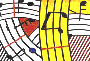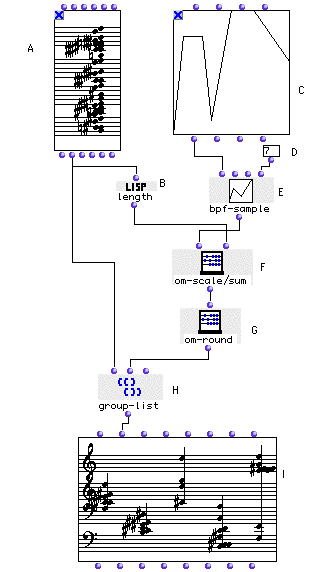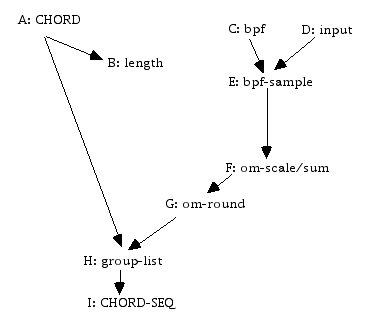Tutorial 31 - Operation on lists II

List grouping into many sub-lists

Topics

BPF, bpf-sample, CHORD, CHORD-SEQ, group-list, length, om-round and om-scale/sum.Description

This example groups a list of notes (A) into subgroups that are defined by a break-point function (C).

Patch structureA: Open the CHORD box (A), enter a list of notes in the "order" mode, close the module and lock it.

B: The length box (B) counts how many notes you have entered in the CHORD box (A).

C: Open the BPF module (C) and edit a curve with the mouse. This curve will be sampled and the samples will correspond to the lengths of the subgroups.

D: In this input (D) you may enter how many samples with which you want the curve to be sampled.

E: The bpf-sample box (E) samples the break-point function (C) using the number of samples defined in the numbox (D).

F: The module om-scaling/sum (F) scales the elements in order to make their sum equal to the number coming out of the in module (B).

G: The module om-round (G) converts the fractional values coming out of the om-scaling/sum box (F) into a list of integers.

H: The module group-list takes the notes coming out of the CHORD box (A) and groups them into subgroups whose lengths are defined by the output of om-scaling/sum (F).

I: Evaluate the CHORD-SEQ box and see the result. Change either the curve in theBPF module, or the value in the input module (D), then re-evaluate the CHORD-SEQ box to see the result.